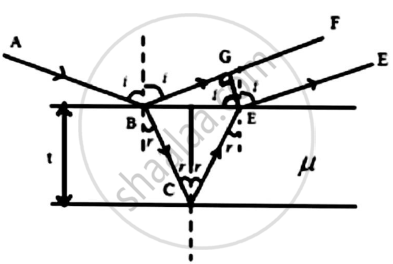# Derive the conditions of maxima and minima due to interference of light reflected from thin film of uniform thickness. - Applied Physics 2

Derive the conditions of maxima and minima due to interference of light reflected from thin film of uniform thickness.

#### SolutionConsider a thin film of uniform thickness (t) and R.I (μ)

On Reflected side,

The ray of light BF and DE will interfere.

The path difference between BF and DE is,
Δ = μ(BC + CD) − BG

BC = CD = t/cosr..........(1)

Now,

BD = (2t) tan r .......(2)

BG = BD sin i

BG = (2t) tan r sin i

BG = 2tμsinr(sinr / cosr)

BG = 2μt(sin^2r/cosr)..........(3)

Substituting (i) and (iii) in Δ :
Δ = μ(t / cosr + t / cosr)−2μt(sin^2r / cosr)
= 2μtcosr(1−sin2r)
Δ = 2μtcosr

This is a geometric path difference. However, there is a phase change of π, as ray BF is reflected from a denser medium. Hence we need to add ±λ2 to path difference

Δ = 2μtcosr ± λ2

For Destructive Interference:

Δ = nλ
2μtcosr±λ2=nλ
2μtcosr=(2n±1)λ2.....(n=0,1,2,...)

This is the required expression for constructive Interference or Maxima.

For Destructive interference:
Δ = (2n±1)λ/2
2μtcosr ± λ/2 = nλ
2μtcosr = nλ

This is the required expression for destructive interference

.

Concept: Interference in Thin Film of Constant Thickness Due to Reflected and Transmitted Light
Is there an error in this question or solution?
2017-2018 (June) CBCGS

Share